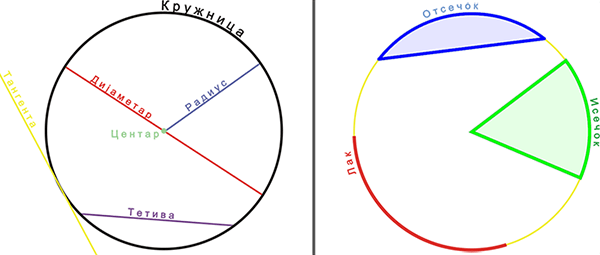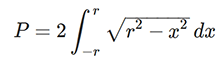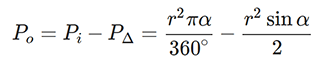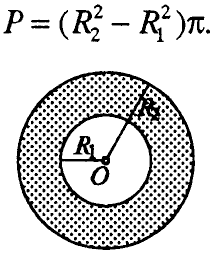# Perimeter of a circle. Area of a circle

## Circle

• The part of the circle bounded by two points lying on it is called a circular arc;
• The part of a circle bounded by a circle and any two semicircles is called a circular section;
• The part of a circle bounded by a circle and one of its tendons is called a circular sectionFig. 1

## 1. Perimeter of a circle and area of a circleFig. 2

## 2. Area of parts of the circle

• The area of a circular section is calculated by the formula:Fig. 3

• Circular arc "l" can be calculated by the following formula:• If we know the circular arc, then the area of the circular section can be calculated with the following formula:• For more advanced mathematicians who can solve integrals, perimeter and area can be calculated using the following formulas:• The area of a circular section can be calculated as the difference between the area of a circular section and the isosceles triangle formed by the two semicircles and the tendon. Given the area of this triangle which, expressed through the known quantities r and α, is:• The area of a circular ring is calculated by the formula:Fig.4

• ## Video lesson – Perimeter of a circle and area of a circle

– perimor na kruznica i plostina na krug –

Total Page Visits: 215 - Today Page Visits: 1